Instantly share code, notes, and snippets.

# simonw/mandelbrot.md Secret

Last active September 10, 2023 00:41
Star You must be signed in to star a gist
Mandelbrot Fractal Visualization

# Mandelbrot Fractal Visualization.

July 30, 2023 at 10:18:59 AM

system:

You are ChatGPT, a large language model trained by OpenAI, based on the GPT-4 architecture. Knowledge cutoff: 2021-09 Current date: 2023-07-30

# Tools

## python

When you send a message containing Python code to python, it will be executed in a stateful Jupyter notebook environment. python will respond with the output of the execution or time out after 120.0 seconds. The drive at '/mnt/data' can be used to save and persist user files. Internet access for this session is disabled. Do not make external web requests or API calls as they will fail.

Prompt:

Draw me a mandelbrot fractal

Generated code:

```import numpy as np
import matplotlib.pyplot as plt

def mandelbrot(c, max_iter):
z = c
for n in range(max_iter):
if abs(z) > 2:
return n
z = z*z + c
return max_iter

def draw_mandelbrot(xmin,xmax,ymin,ymax,width,height,max_iter):
r1 = np.linspace(xmin, xmax, width)
r2 = np.linspace(ymin, ymax, height)
return (r1,r2,np.array([[mandelbrot(complex(r, i),max_iter) for r in r1] for i in r2]))

def draw_image(xmin,xmax,ymin,ymax,width,height,max_iter):
d = draw_mandelbrot(xmin,xmax,ymin,ymax,width,height,max_iter)
plt.imshow(d, extent=(xmin, xmax, ymin, ymax))
plt.show()

draw_image(-2.0,1.0,-1.5,1.5,1000,1000,256)```

Execution output: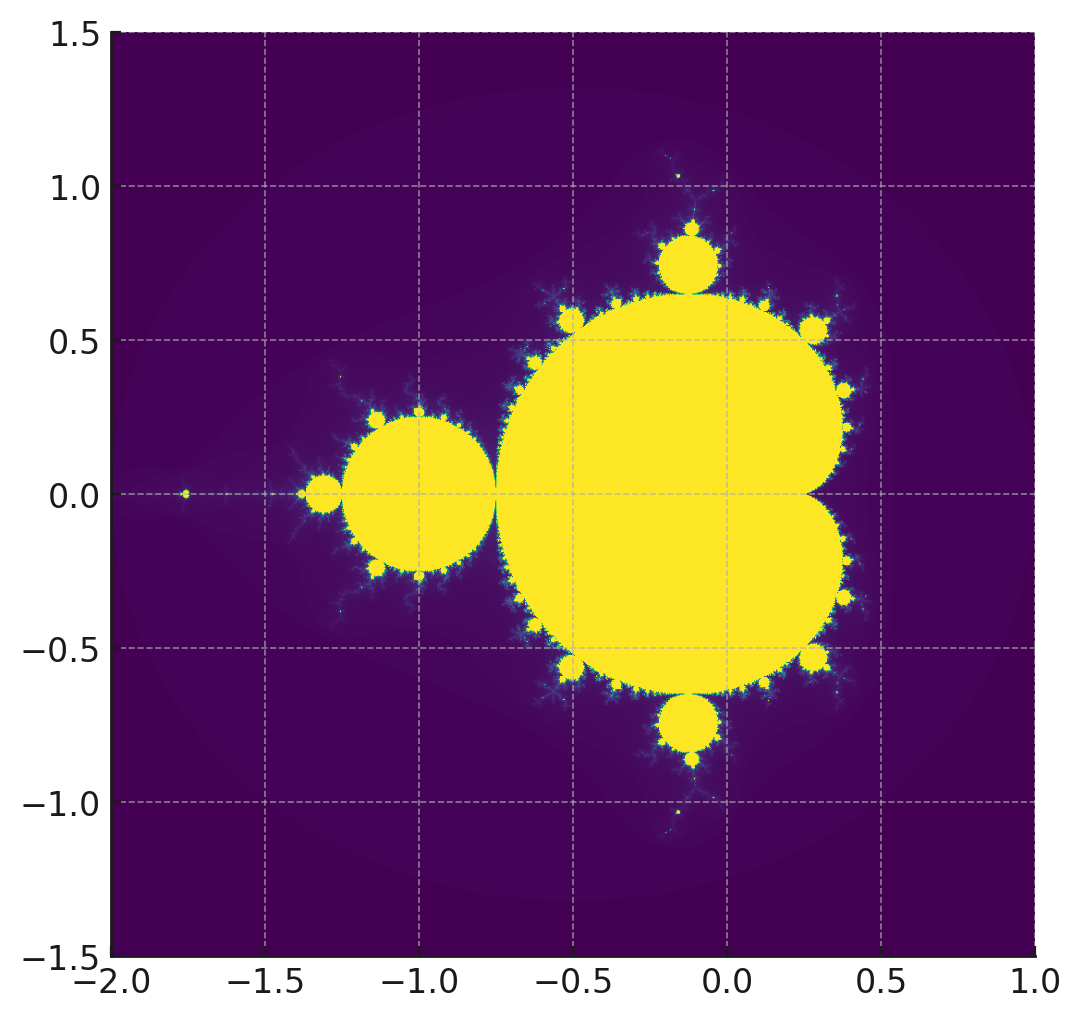ChatGPT:

Here is a visual representation of the Mandelbrot set, a popular example of a fractal in mathematics. This set is defined as the set of complex numbers (c) for which the function (f_c(z) = z^2 + c) does not diverge when iterated from (z = 0).

Prompt:

Zoom in on 2x 0.0 y, -1.275 x and draw it again

Generated code:

```# Defining the new zoomed-in coordinates
zoom_xmin = -1.275 - 1.0
zoom_xmax = -1.275 + 1.0
zoom_ymin = 0.0 - 1.0
zoom_ymax = 0.0 + 1.0

# Drawing the zoomed-in Mandelbrot fractal
draw_image(zoom_xmin, zoom_xmax, zoom_ymin, zoom_ymax, 1000, 1000, 256)```

Execution output: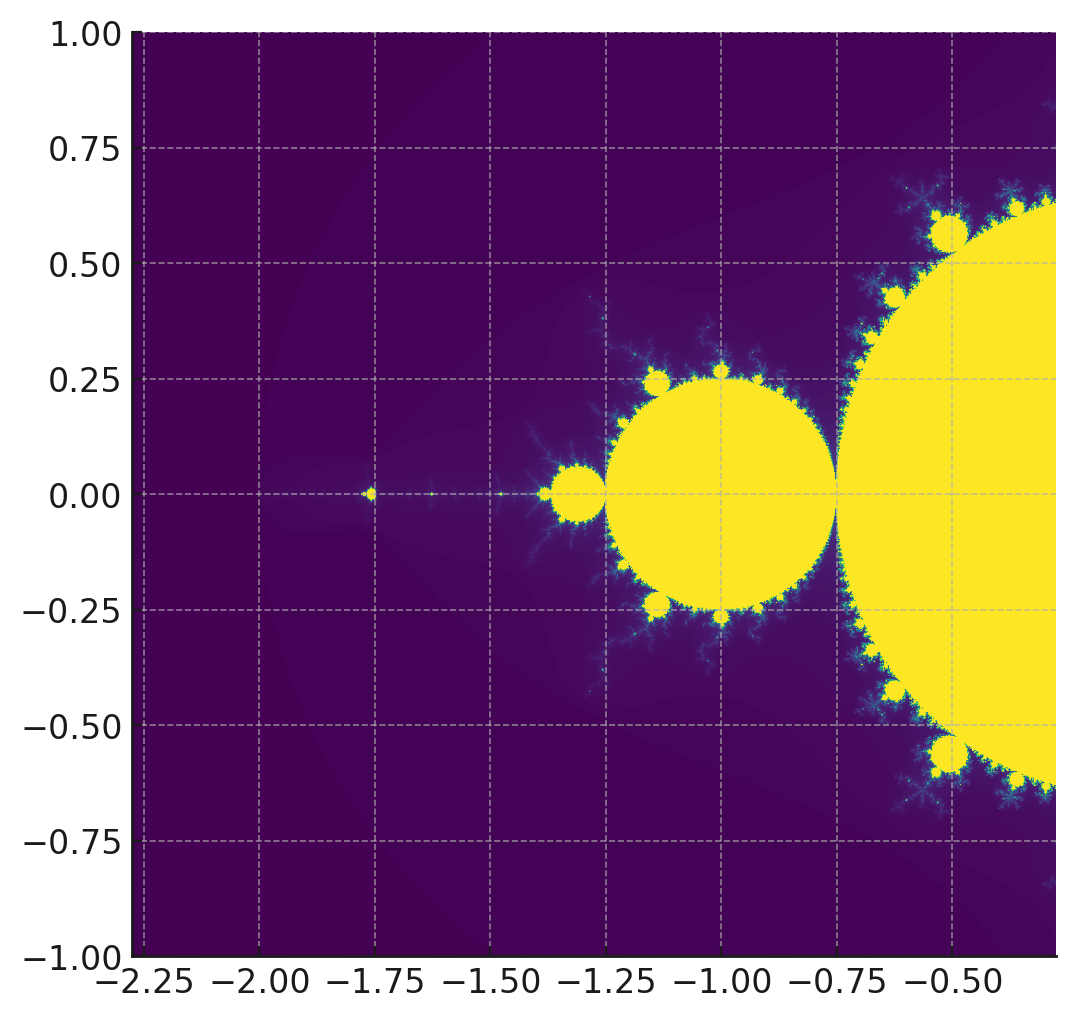ChatGPT:

Here's a zoomed-in view of the Mandelbrot set, centered around ( x = -1.275 ) and ( y = 0.0 ), with a 2x zoom. The intricate details and complex patterns within the fractal become more apparent as you zoom in on specific regions.

Prompt:

Save the first two images to disk. Then zoom in on the second image using the same center four more times, saving each image

Generated code:

```# Function to save the Mandelbrot image
def save_image(xmin, xmax, ymin, ymax, width, height, max_iter, filename):
d = draw_mandelbrot(xmin, xmax, ymin, ymax, width, height, max_iter)
plt.imshow(d, extent=(xmin, xmax, ymin, ymax))
plt.savefig(filename)

# Saving the initial image
save_image(-2.0, 1.0, -1.5, 1.5, 1000, 1000, 256, "/mnt/data/mandelbrot_initial.png")

# Saving the first zoomed-in image
save_image(zoom_xmin, zoom_xmax, zoom_ymin, zoom_ymax, 1000, 1000, 256, "/mnt/data/mandelbrot_zoom1.png")

# Zooming in 4 more times, and saving each image
for i in range(2, 6):
zoom_factor = 2**i
new_xmin = -1.275 - 1.0 / zoom_factor
new_xmax = -1.275 + 1.0 / zoom_factor
new_ymin = 0.0 - 1.0 / zoom_factor
new_ymax = 0.0 + 1.0 / zoom_factor
save_image(new_xmin, new_xmax, new_ymin, new_ymax, 1000, 1000, 256, f"/mnt/data/mandelbrot_zoom{i}.png")

# List of saved files
saved_files = [f"/mnt/data/mandelbrot_zoom{i}.png" for i in range(6)]
saved_files = "/mnt/data/mandelbrot_initial.png"
saved_files```

Execution output:

``````---------------------------------------------------------------------------
KeyboardInterrupt                         Traceback (most recent call last)
Cell In, line 20
18     new_ymin = 0.0 - 1.0 / zoom_factor
19     new_ymax = 0.0 + 1.0 / zoom_factor
---> 20     save_image(new_xmin, new_xmax, new_ymin, new_ymax, 1000, 1000, 256, f"/mnt/data/mandelbrot_zoom{i}.png")
22 # List of saved files
23 saved_files = [f"/mnt/data/mandelbrot_zoom{i}.png" for i in range(6)]

Cell In, line 3, in save_image(xmin, xmax, ymin, ymax, width, height, max_iter, filename)
2 def save_image(xmin, xmax, ymin, ymax, width, height, max_iter, filename):
----> 3     d = draw_mandelbrot(xmin, xmax, ymin, ymax, width, height, max_iter)
4     plt.imshow(d, extent=(xmin, xmax, ymin, ymax))
5     plt.savefig(filename)

Cell In, line 15, in draw_mandelbrot(xmin, xmax, ymin, ymax, width, height, max_iter)
13 r1 = np.linspace(xmin, xmax, width)
14 r2 = np.linspace(ymin, ymax, height)
---> 15 return (r1,r2,np.array([[mandelbrot(complex(r, i),max_iter) for r in r1] for i in r2]))

Cell In, line 15, in <listcomp>(.0)
13 r1 = np.linspace(xmin, xmax, width)
14 r2 = np.linspace(ymin, ymax, height)
---> 15 return (r1,r2,np.array([[mandelbrot(complex(r, i),max_iter) for r in r1] for i in r2]))

Cell In, line 15, in <listcomp>(.0)
13 r1 = np.linspace(xmin, xmax, width)
14 r2 = np.linspace(ymin, ymax, height)
---> 15 return (r1,r2,np.array([[mandelbrot(complex(r, i),max_iter) for r in r1] for i in r2]))

Cell In, line 7, in mandelbrot(c, max_iter)
5 z = c
6 for n in range(max_iter):
----> 7     if abs(z) > 2:
8         return n
9     z = z*z + c

KeyboardInterrupt:

WARNING: Automatic interrupt triggered after 60.0 seconds.
``````

ChatGPT:

It seems that the computation of the zoomed-in images is taking too long, and the operation was interrupted due to the time limit. Generating these fractal images can be computationally expensive, especially as we zoom in further.

Let's try a different approach by reducing the resolution and increasing the number of iterations for the zoomed-in images. This should give us a better balance between detail and computation time.

Generated code:

```# Reducing resolution and increasing iterations for zoomed-in images
width = 500
height = 500
max_iter = 512

# Saving the first zoomed-in image again
save_image(zoom_xmin, zoom_xmax, zoom_ymin, zoom_ymax, width, height, max_iter, "/mnt/data/mandelbrot_zoom1.png")

# Zooming in 4 more times, and saving each image
for i in range(2, 6):
zoom_factor = 2**i
new_xmin = -1.275 - 1.0 / zoom_factor
new_xmax = -1.275 + 1.0 / zoom_factor
new_ymin = 0.0 - 1.0 / zoom_factor
new_ymax = 0.0 + 1.0 / zoom_factor
save_image(new_xmin, new_xmax, new_ymin, new_ymax, width, height, max_iter, f"/mnt/data/mandelbrot_zoom{i}.png")

# List of saved files
saved_files_zoomed = [f"/mnt/data/mandelbrot_zoom{i}.png" for i in range(1, 6)]
saved_files_zoomed```

Execution output:

``````---------------------------------------------------------------------------
KeyboardInterrupt                         Traceback (most recent call last)
Cell In, line 16
14     new_ymin = 0.0 - 1.0 / zoom_factor
15     new_ymax = 0.0 + 1.0 / zoom_factor
---> 16     save_image(new_xmin, new_xmax, new_ymin, new_ymax, width, height, max_iter, f"/mnt/data/mandelbrot_zoom{i}.png")
18 # List of saved files
19 saved_files_zoomed = [f"/mnt/data/mandelbrot_zoom{i}.png" for i in range(1, 6)]

Cell In, line 3, in save_image(xmin, xmax, ymin, ymax, width, height, max_iter, filename)
2 def save_image(xmin, xmax, ymin, ymax, width, height, max_iter, filename):
----> 3     d = draw_mandelbrot(xmin, xmax, ymin, ymax, width, height, max_iter)
4     plt.imshow(d, extent=(xmin, xmax, ymin, ymax))
5     plt.savefig(filename)

Cell In, line 15, in draw_mandelbrot(xmin, xmax, ymin, ymax, width, height, max_iter)
13 r1 = np.linspace(xmin, xmax, width)
14 r2 = np.linspace(ymin, ymax, height)
---> 15 return (r1,r2,np.array([[mandelbrot(complex(r, i),max_iter) for r in r1] for i in r2]))

Cell In, line 15, in <listcomp>(.0)
13 r1 = np.linspace(xmin, xmax, width)
14 r2 = np.linspace(ymin, ymax, height)
---> 15 return (r1,r2,np.array([[mandelbrot(complex(r, i),max_iter) for r in r1] for i in r2]))

Cell In, line 15, in <listcomp>(.0)
13 r1 = np.linspace(xmin, xmax, width)
14 r2 = np.linspace(ymin, ymax, height)
---> 15 return (r1,r2,np.array([[mandelbrot(complex(r, i),max_iter) for r in r1] for i in r2]))

Cell In, line 9, in mandelbrot(c, max_iter)
7     if abs(z) > 2:
8         return n
----> 9     z = z*z + c
10 return max_iter

KeyboardInterrupt:

WARNING: Automatic interrupt triggered after 60.0 seconds.
``````

ChatGPT:

It appears that the calculations are still taking too long, even after reducing the resolution and increasing the number of iterations. This is likely due to the increasing complexity of the fractal as we zoom in further.

We can try to solve this issue by further reducing the resolution and using a more optimized approach to generate the images. Let's proceed by taking these measures.

Generated code:

```from numba import jit

# Optimizing the mandelbrot function using Just-In-Time (JIT) compilation
@jit
def mandelbrot(c, max_iter):
z = c
for n in range(max_iter):
if abs(z) > 2:
return n
z = z*z + c
return max_iter

# Reducing the resolution for zoomed-in images
width_zoom = 300
height_zoom = 300

# Saving the first zoomed-in image again
save_image(zoom_xmin, zoom_xmax, zoom_ymin, zoom_ymax, width_zoom, height_zoom, max_iter, "/mnt/data/mandelbrot_zoom1.png")

# Zooming in 4 more times, and saving each image
for i in range(2, 6):
zoom_factor = 2**i
new_xmin = -1.275 - 1.0 / zoom_factor
new_xmax = -1.275 + 1.0 / zoom_factor
new_ymin = 0.0 - 1.0 / zoom_factor
new_ymax = 0.0 + 1.0 / zoom_factor
save_image(new_xmin, new_xmax, new_ymin, new_ymax, width_zoom, height_zoom, max_iter, f"/mnt/data/mandelbrot_zoom{i}.png")

# List of saved files including the initial image
saved_files_optimized = [f"/mnt/data/mandelbrot_zoom{i}.png" for i in range(1, 6)]
saved_files_optimized.insert(0, "/mnt/data/mandelbrot_initial.png")
saved_files_optimized```

Execution output:

``````/tmp/ipykernel_32/3951458685.py:5: NumbaDeprecationWarning: The 'nopython' keyword argument was not supplied to the 'numba.jit' decorator. The implicit default value for this argument is currently False, but it will be changed to True in Numba 0.59.0. See https://numba.readthedocs.io/en/stable/reference/deprecation.html#deprecation-of-object-mode-fall-back-behaviour-when-using-jit for details.
def mandelbrot(c, max_iter):
``````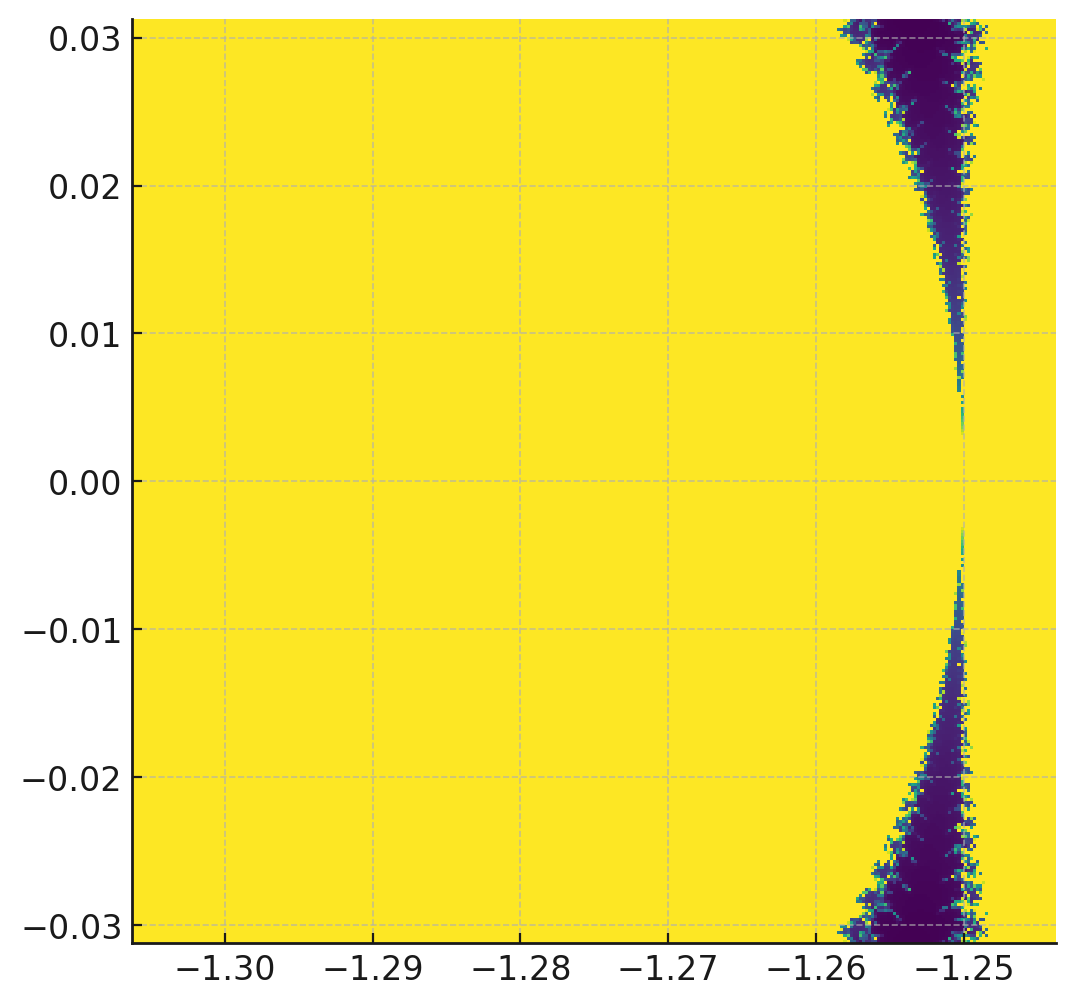ChatGPT:

The Mandelbrot images have been successfully generated and saved, including the initial image and five zoomed-in images. Here are the links to download the images:

1. Initial Mandelbrot Image
2. Zoom Level 1
3. Zoom Level 2
4. Zoom Level 3
5. Zoom Level 4
6. Zoom Level 5

Prompt:

Stitch those images together into an animated GIF, 0.5s per frame

Generated code:

```from PIL import Image

# List to hold the frames of the GIF
frames = []

for file in saved_files_optimized:
frame = Image.open(file)
frames.append(frame)

# Saving the frames as an animated GIF
gif_path = "/mnt/data/mandelbrot_animation.gif"
frames.save(gif_path, save_all=True, append_images=frames[1:], duration=500, loop=0)

# Path to the animated GIF
gif_path```

Execution output:

``````'/mnt/data/mandelbrot_animation.gif'
``````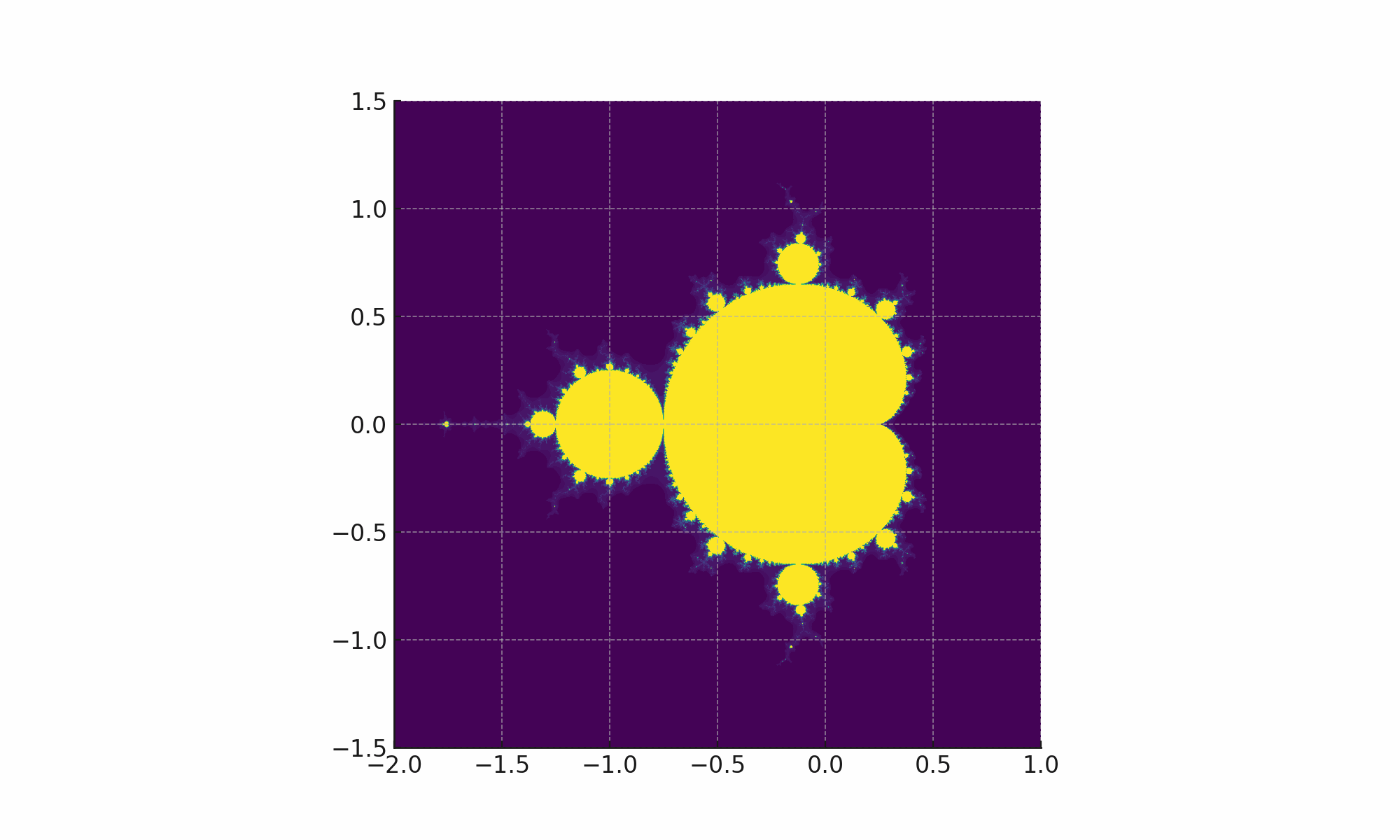ChatGPT:

The Mandelbrot images have been stitched together into an animated GIF, with a duration of 0.5 seconds per frame. You can download and view the animated GIF by clicking the link below:

Prompt:

that fractal is mostly yellow, can you make it so there are more interesting colors throughout?

ChatGPT:

Certainly! The colors in the visualization of the Mandelbrot set are determined by the mapping of iteration counts to a color palette. By using a more varied color palette, we can create a more visually interesting image.

Let's modify the color mapping and generate the animated GIF again.

Generated code:

```from matplotlib.colors import hsv_to_rgb

def draw_mandelbrot_color(xmin, xmax, ymin, ymax, width, height, max_iter):
r1 = np.linspace(xmin, xmax, width)
r2 = np.linspace(ymin, ymax, height)
image = np.empty((height, width, 3))
for y, i in enumerate(r2):
for x, r in enumerate(r1):
c = complex(r, i)
z = c
for n in range(max_iter):
if abs(z) > 2:
break
z = z * z + c
# Using HSV color space to create a colorful pattern
ratio = n / max_iter
hue = 360 * ratio
saturation = 1.0
value = 1.0 if n < max_iter else 0
color = hsv_to_rgb((hue / 360, saturation, value))
image[y, x] = color
return image

def save_image_color(xmin, xmax, ymin, ymax, width, height, max_iter, filename):
image = draw_mandelbrot_color(xmin, xmax, ymin, ymax, width, height, max_iter)
plt.imsave(filename, image)

# Saving the initial and zoomed-in images with colorful pattern
for i, file in enumerate(saved_files_optimized):
if i == 0:
save_image_color(-2.0, 1.0, -1.5, 1.5, width, height, max_iter, file)
else:
zoom_factor = 2**i
new_xmin = -1.275 - 1.0 / zoom_factor
new_xmax = -1.275 + 1.0 / zoom_factor
new_ymin = 0.0 - 1.0 / zoom_factor
new_ymax = 0.0 + 1.0 / zoom_factor
save_image_color(new_xmin, new_xmax, new_ymin, new_ymax, width_zoom, height_zoom, max_iter, file)

# Creating animated GIF with colorful images
frames_color = [Image.open(file) for file in saved_files_optimized]
gif_path_color = "/mnt/data/mandelbrot_animation_color.gif"
frames_color.save(gif_path_color, save_all=True, append_images=frames_color[1:], duration=500, loop=0)

# Path to the colorful animated GIF
gif_path_color```

Execution output:

``````---------------------------------------------------------------------------
KeyboardInterrupt                         Traceback (most recent call last)
Cell In, line 38
36         new_ymin = 0.0 - 1.0 / zoom_factor
37         new_ymax = 0.0 + 1.0 / zoom_factor
---> 38         save_image_color(new_xmin, new_xmax, new_ymin, new_ymax, width_zoom, height_zoom, max_iter, file)
40 # Creating animated GIF with colorful images
41 frames_color = [Image.open(file) for file in saved_files_optimized]

Cell In, line 25, in save_image_color(xmin, xmax, ymin, ymax, width, height, max_iter, filename)
24 def save_image_color(xmin, xmax, ymin, ymax, width, height, max_iter, filename):
---> 25     image = draw_mandelbrot_color(xmin, xmax, ymin, ymax, width, height, max_iter)
26     plt.imsave(filename, image)

Cell In, line 14, in draw_mandelbrot_color(xmin, xmax, ymin, ymax, width, height, max_iter)
12     if abs(z) > 2:
13         break
---> 14     z = z * z + c
15 # Using HSV color space to create a colorful pattern
16 ratio = n / max_iter

KeyboardInterrupt:

WARNING: Automatic interrupt triggered after 60.0 seconds.
``````

ChatGPT:

It appears that the coloring process is still taking too long. We can optimize the code by using NumPy to handle array operations more efficiently. Let's make the necessary adjustments and try again.

Generated code:

```def mandelbrot_color(c, max_iter):
z = c
for n in range(max_iter):
if abs(z) > 2:
# Using HSV color space to create a colorful pattern
ratio = n / max_iter
hue = 360 * ratio
saturation = 1.0
value = 1.0 if n < max_iter else 0
return hsv_to_rgb((hue / 360, saturation, value))
z = z * z + c
return (0, 0, 0)

def draw_mandelbrot_color(xmin, xmax, ymin, ymax, width, height, max_iter):
r1 = np.linspace(xmin, xmax, width)
r2 = np.linspace(ymin, ymax, height)
return np.array([[mandelbrot_color(complex(r, i), max_iter) for r in r1] for i in r2])

# Saving the initial and zoomed-in images with colorful pattern
for i, file in enumerate(saved_files_optimized):
if i == 0:
save_image_color(-2.0, 1.0, -1.5, 1.5, width, height, max_iter, file)
else:
zoom_factor = 2**i
new_xmin = -1.275 - 1.0 / zoom_factor
new_xmax = -1.275 + 1.0 / zoom_factor
new_ymin = 0.0 - 1.0 / zoom_factor
new_ymax = 0.0 + 1.0 / zoom_factor
save_image_color(new_xmin, new_xmax, new_ymin, new_ymax, width_zoom, height_zoom, max_iter, file)

# Creating animated GIF with colorful images
frames_color = [Image.open(file) for file in saved_files_optimized]
gif_path_color = "/mnt/data/mandelbrot_animation_color.gif"
frames_color.save(gif_path_color, save_all=True, append_images=frames_color[1:], duration=500, loop=0)

# Path to the colorful animated GIF
gif_path_color```

Execution output:

``````'/mnt/data/mandelbrot_animation_color.gif'
``````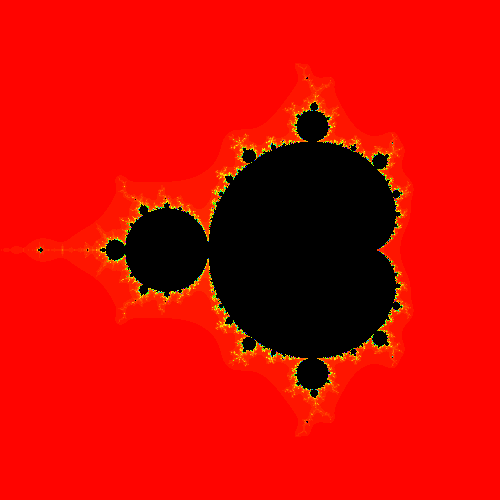ChatGPT:

The Mandelbrot images have been recreated with a colorful pattern and stitched together into an animated GIF. You can download and view the new colorful animated GIF by clicking the link below: